International
Tables for
Crystallography
Volume C
Mathematical, physical and chemical tables
Edited by E. Prince

International Tables for Crystallography (2006). Vol. C, ch. 6.4, p. 611

## Section 6.4.10. The extinction factor

T. M. Sabinea

aANSTO, Private Mail Bag 1, Menai, NSW 2234, Australia

### 6.4.10. The extinction factor

| top | pdf |

#### 6.4.10.1. The correlated block model

| top | pdf |

For this model of the real crystal, the variable x is given by equation (6.4.8.6), withand g the refinable variables. Extinction factors are then calculated from equations (6.4.5.3), (6.4.5.4), and (6.4.5.5). For a reflection at a scattering angle offrom a reasonably equiaxial crystal, the appropriate extinction factor is given by (6.4.7.1)as E(2θ) =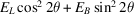.

It is a meaningful procedure to refine both primary and secondary extinction in this model. The reason for the high correlation betweenand g that is found when other theories are applied, for example that of Becker & Coppens (1974), lies in the structure of the quantity x. In the theory presented here, x is proportional to F2 for pure primary extinction and to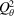for pure secondary extinction.

#### 6.4.10.2. The uncorrelated block model

| top | pdf |

When this model is used, two values of x are required. These are designated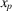for primary extinction and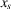for secondary extinction. Equation (6.4.8.1)is used to obtain a value for. The primary-extinction factors are then calculated from (6.4.5.3), (6.4.5.4)and (6.4.5.5), and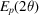is given by equation (6.4.7.1). In the second step,is obtained from equation (6.4.9.1), and the secondary-extinction factors are calculated from either (6.4.9.2)and (6.4.9.3)or (6.4.9.4)and (6.4.9.5). The result of these calculations is then used in equation (6.4.7.1)to give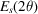. It is emphasised thatincludes the primary-extinction factor. Finally,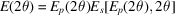.

Application of both models to the analysis of neutron diffraction data has been carried out by Kampermann, Sabine, Craven & McMullan (1995).

### References

Becker, P. J. & Coppens, P. (1974). Extinction within the limit of validity of the Darwin transfer equations. I. General formalisms for primary and secondary extinction and their application to spherical crystals. Acta Cryst. A30, 129–147.
Kampermann, S. P., Sabine, T. M., Craven, B. M. & McMullan, R. K. (1995). Hexamethylenetetramine: extinction and thermal vibrations from neutron diffraction at six temperatures. Acta Cryst. A51, 489–497.